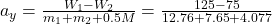## Two weights are connected by a very light cord that passes over an 80.0 N frictionless pulley of radius 0.300 m. The pulley is a solid unifo

Question

Two weights are connected by a very light cord that passes over an 80.0 N frictionless pulley of radius 0.300 m. The pulley is a solid uniform disk and is supported by a hook connected to the ceiling. What force does the ceiling exert on the hook

in progress 0
6 months 2021-07-21T02:06:50+00:00 1 Answers 2 views 0

The force the ceiling  exert on the hook is 321.67 N

Explanation:

Here we have the weights being 75 N and 125 N

The weight of the pulley = 80 N

Total weight hung from the ceiling = 75 + 125 + 80 = 280 N

Therefore since the forces acting on the ceiling tends to act downwards as they are the forces due to weights, we have

The force on the

T₁ – 125 = m₂a =

m₂ = 125/9.8 = 12.76 kg

Similarly T₂ – 75 = m₁a, m₁ = 7.65 kg

acceleration is given by= 2.042 m/s²

Therefore

T₁ – 125 = m₂a = 12.76 × 2.042 = 26.04 N and

T₁ = 125 + 26.04 = 151.04 N

Similarly T₂ – 75 = m₁a =  7.65 × 2.042  = 15.63 N

T₂ = 15.63  + 75 = 90.63 N

Force on hook = Force exerted by ceiling on hook = T₁ + T₂ + Weight of pulley = 151.04  + 90.63 + 80  = 321.67 N